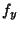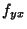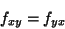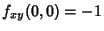## Partial Derivative

Partial derivatives are defined as derivatives of a function of multiple variables when all but the variable of interest are held fixed during the differentiation.(1)

The above partial derivative is sometimes denotedfor brevity. For a nice'' 2-D function(i.e., one for which,,,,exist and are continuous in a Neighborhood), then. Partial derivatives involving more than one variable are called Mixed Partial Derivatives.

For nice functions, mixed partial derivatives must be equal regardless of the order in which the differentiation is performed so, for example,(2)(3)

For an Exact Differential,(4)

so(5)

If the continuity requirement for Mixed Partials is dropped, it is possible to construct functions for which Mixed Partials are not equal. An example is the function(6)

which hasand(Wagon 1991). This function is depicted above and by Fischer (1986).

Abramowitz and Stegun (1972) give Finite Difference versions for partial derivatives.

References

Abramowitz, M. and Stegun, C. A. (Eds.). Handbook of Mathematical Functions with Formulas, Graphs, and Mathematical Tables, 9th printing. New York: Dover, pp. 883-885, 1972.

Fischer, G. (Ed.). Plate 121 in Mathematische Modelle/Mathematical Models, Bildband/Photograph Volume. Braunschweig, Germany: Vieweg, p. 118, 1986.

Thomas, G. B. and Finney, R. L. §16.8 in Calculus and Analytic Geometry, 9th ed. Reading, MA: Addison-Wesley, 1996.

Wagon, S. Mathematica in Action. New York: W. H. Freeman, pp. 83-85, 1991.